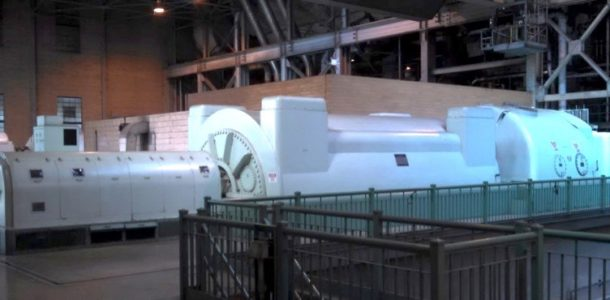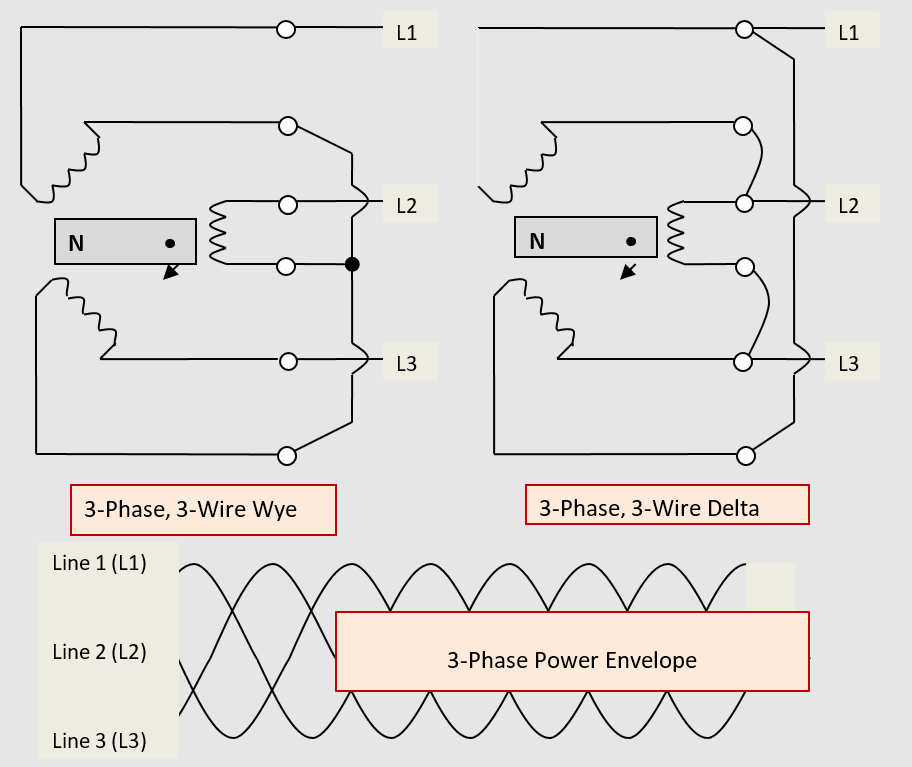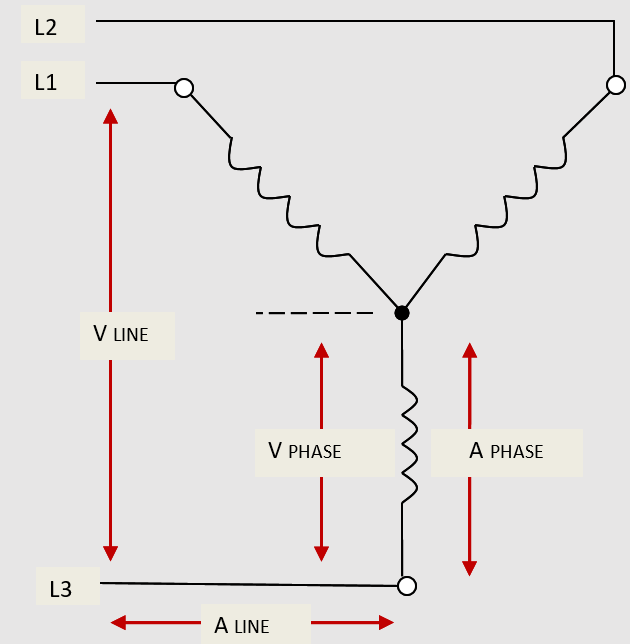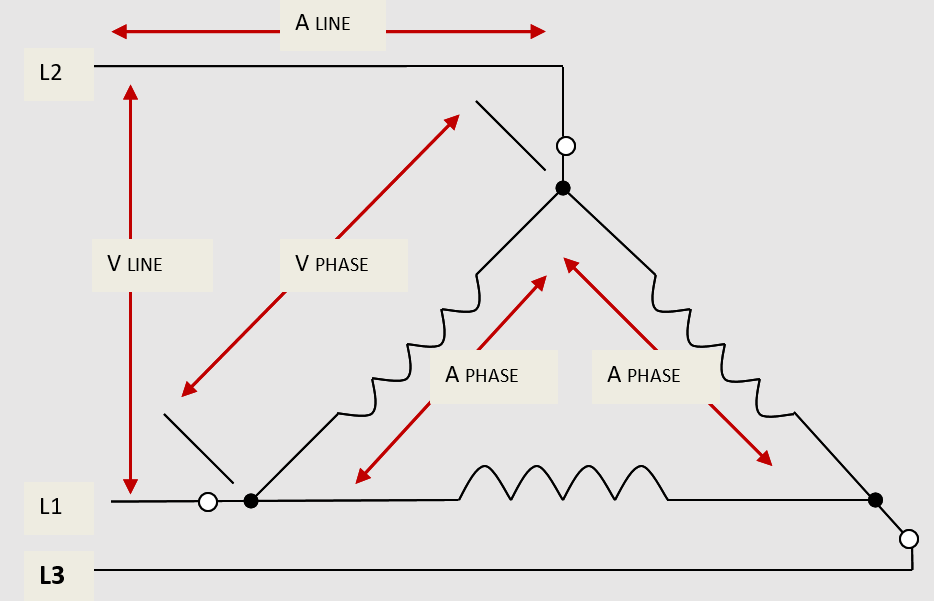Home / Electric Power / Three-Phase AC Power: Star & Delta Connection

# Three-Phase AC Power: Star & Delta Connection

Want create site? Find Free WordPress Themes and plugins.

The most economical way to mass produce AC power is by steam turbines that drive large three-phase AC generators (alternators — Figure 1).Figure 1. Steam turbines drive large 3-phase AC generators

This is why most electrical-utility power generating plants are located on a river that has been dammed to form a lake. Before electricity was mass produced by large steam turbines, the fall of the water from the dam was used to turn the turbines to generate hydroelectric power as shown in Figure 2.Figure 2. Piedmont Hydro Project, Saluda River

As shown in Figure 3, three single-phase AC sources can be produced in the same alternator from a common rotating electromagnetic field.

The 120 mechanical-degree displacement within the alternator generates an electrical displacement of 120 electrical degrees between any two adjacent single-phase AC sources: the single-phase AC source at the top of the drawing leads the single-phase AC source in the middle of the drawing by 120 electrical degrees.

The single-phase AC source in the middle of the drawing leads the single-phase AC source at the bottom of the drawing by 120 electrical degrees.

If the sine waveforms for each single-phase AC source were extended along the width of the page, it would be shown that the single-phase AC source at the bottom of the drawing also leads the single-phase AC source at the top of the drawing by 120 electrical degrees.

When six conductors, two for each single-phase AC source, are brought out from the alternator and extended to three individually-connected single-phase AC loads, there is no interaction between the single-phase AC sources, only phase displacement.Figure 3. Generation of three single-phase AC 2-wire sources from a common magnetic rotor circuit

As shown in Figure 4, the winding leads of the three single-phase AC, 2-wire sources can be interconnected at the alternator for transmission of the 3-phase AC power using only three circuit conductors (or transmission lines) instead of the six leads shown in Figure 3.

The 3-phase AC alternator (or motor or transformer) is connected in a 3-phase delta configuration when the respective ends of the individual single-phase windings are connected in a closed-loop series configuration and the common-end connection of any two adjacent windings is brought out as one of the three 3-phase AC line conductors.

The 3-phase alternator (or motor or transformer) is connected in a 3-phase wye configuration when the same-reference ends (leads) of each individual single-phase winding are connected to form a common point, and the other winding ends (brought out as the three 3-phase AC line conductors) appear to be in parallel. The three 3-phase AC line conductors are normally identified as Line 1 (L1) or phase A, Line 2 (L2) or phase B, and Line 3 (L3) or phase C.Figure 4. Generation of 3-phase AC 3-wire power

Three-phase AC power is the more common form of electrical power generation, transmission, and distribution, simply because the 3-phase power envelope (again, Figure 4) never goes through a zero power transition as each of the three contained single-phase AC sources do.

The direction of current flow in an electrical circuit is always from a negative-voltage potential to a positive voltage potential.

In a 3-phase wye or delta wiring configuration, when any one single-phase AC source goes through its zero-volt transition, current flow is between the other two single-phase AC sources. When all three single-phase AC sources are in alternation (producing either positive or negative voltage), current flow is still between a negative-voltage potential and a positive-voltage potential:

According to the time frame, the 3-phase AC line current may be flowing from the first single-phase AC source (L1) to both of the other single-phase AC sources (both L2 and L3), and a moment later, the 3-phase AC line current may be flowing from the second single-phase AC source to the other two, and so on.

Whether to connect the three single-phase windings in a 3-phase AC alternator (or motor or transformer) in a wye or delta wiring configuration depends on the desired magnitude of the line voltage compared to the desired magnitude of the line current.

Coming out of the alternator or transformer, the 3-phase wye wiring configuration is referred to as a voltage multiplier. Going into a 3-phase motor or transformer, the 3-phase wye wiring configuration is referred to as a voltage divider.

In comparison, coming out of the alternator or transformer, the 3-phase delta wiring configuration is referred to as a current multiplier. Going into a 3-phase motor or transformer, the 3-phase delta wiring configuration is referred to as a current divider.

## Three-Phase Wye (Star) Connection

As shown in Figure 5, in the 3-phase wye (star) connection, the three single-phase AC alternator windings (or motor or transformer windings) are wired in parallel with the same-reference lead of each winding connected (one each) to supply conductors L1 (line 1), L2 (line 2), and L3 (line 3). The other ends or leads of the three single-phase windings are connected commonly to each other.Figure 5. Voltage-current relationships in a 3-phase wye wiring configuration

The 3-phase wye wiring configuration is referred to as a voltage multiplier/divider.  This reference comes from the 3-phase AC line voltage [normally referred to as either the “line-to-line voltage” or just “line voltage” (V line)].  It is measured between any two of the three supply lines in the 3-phase alternator (or motor or transformer) wye wiring configuration.

It is then applied as the sum of the two individual single-phase AC voltage drops (V phase) across any two of the three single-phase windings.

Although as shown in Figure 5, these two windings are effectively wired in series (in reference to the points of voltage measure), the two single-phase AC winding voltages do not add algebraically (straight addition — which would equal two times the voltage of either winding). The respective rated sinusoidal voltages of the two single-phase windings are 120 electrical degrees out-of-phase with each other.  Because of this 1200 “phase-shift”, the two single-phase AC winding voltages add vectorially (as phasor quantities).

In Figure 5, the 3-phase AC line voltage is shown as measured from Line 1 to Line 3. If the single-phase winding voltage of all three of the single-phase AC source windings in this 3-phase alternator equals 277 volts: The vector addition of the two single-phase AC winding voltages equals:

Line 1:  SIN of 1200 × 277 volts = 0.866 × 277 volts = 240 volts

Line 3:  SIN of 1200 × 277 volts = 0.866 × 277 volts = 240 volts

1.732 × 277 volts = 480 volts

When the two 240-volt measures are added together, the line voltage of the 3-phase alternator connected in a wye wiring configuration equals 480 volts. The sine function of 1200 is equal to 0.866.

Since the line voltage must be measured as the line-to-line voltage between any two of the three single-phase AC sources in the alternator (or motor or transformer), the sum of 0.866 plus 0.866, which is equal to 1.732, can be multiplied times the 277-volt rating of either winding to obtain the same 480-volt line-voltage rating.

By coincidence or luck, the square-root of 3 (√3) is also equal to 1.732, such that mastering the math skills of trigonometry is not required. Just remember when determining the 3-phase AC line voltage of a wye-connected alternator (motor or transformer) that the line voltage is equal to 1.732 times the phase voltage, or:

The 3-phase AC line voltage in a wye wiring configuration is 173% (√3) greater than the single-phase AC winding voltage.

In formula:

VLINE = √3 × VPHASE (WINDING)

Or       VPHASE = VLINE ÷ √3

In comparison, the magnitudes of the 3-phase AC line current and the single-phase AC winding current are equal in a wye wiring configuration. The start of each respective single-phase winding in Figure 5 is connected directly to one of the line conductors (lead L1, L2, or L3), such that all of the 3-phase AC line current (A line) of that phase passes through the coil of that single-phase winding;

ALINE = APHASE (WINDING)

From the common connection of the other ends or leads of the three single-phase windings, the single-phase AC current of a given phase or winding divides between the other two phases or windings.

## Three-Phase Delta Connection

As shown in Figure 6, the 3-phase delta connection is simply a closed-loop circuit in which the three single-phase alternator windings are wired in series with each other.

The out (or end) lead of one single-phase winding is connected to the in the lead of the second single-phase winding. The out lead of the second single-phase winding is connected to the in the lead of the third single-phase winding. The out lead of the third single-phase winding is connected to the in the lead of the first single-phase winding: Supply lines L1, L2, and L3 are connected, one each, to the three in-out winding-lead node connections.Figure 6. Voltage-current relationships in a 3-phase delta wiring configuration

When the individual single-phase winding leads [beginning (in) and ending (out) leads or terminals of each respective single-phase winding] of a 3-phase AC alternator (motor or transformer) are connected in a delta configuration, each single-phase winding is connected directly across two of the three supply lines.  When this occurs, the 3-phase AC line voltage measured between any two supply lines will be equal to the single-phase AC voltage measured across the same in-out winding nodes (connections).

In Figure 6, the 3-phase AC line voltage measured between supply lines L1 and L2 is equal to the single-phase AC winding voltage measured across the in-out nodes connected to L1 and L2:

The magnitudes of the 3-phase AC line voltage and the single-phase AC winding voltage are equal in a delta configuration.

In formula:

VLINE = VPHASE (WINDING)

In comparison, the 3-phase AC line current supplied to any one of the three supply conductors in the delta alternator configuration is a composite of the AC currents flowing from the two single-phase windings connected to that line by their respective in-out nodes.

Said another way, the two single-phase AC line currents flowing from the other two 3-phase line conductors into a given in-out node, have a common line-conductor path out of the node. Although these two windings are effectively wired in parallel to the single line conductor, all three single-phase AC winding currents are 120 electrical degrees out-of-phase with each other, such that

ALINE = √3 × APHASE (WINDING)

Or       APHASE = ALINE ÷ √3

Because of the 1200 phase shift between any two adjacent winding currents, the single-phase AC winding currents add vectorially (phasor quantities — in the same manner as discussed for the voltages in a wye configuration), not algebraically (the √3 used in these calculations is equal to 1.732).

If the two single-phase AC winding currents were added algebraically, the composite 3-phase AC line current would be equal to two times the single-phase AC (winding) current value.

Did you find apk for android? You can find new Free Android Games and apps.

### About Ahmad FaizanMr. Ahmed Faizan Sheikh, M.Sc. (USA), Research Fellow (USA), a member of IEEE & CIGRE, is a Fulbright Alumnus and earned his Master’s Degree in Electrical and Power Engineering from Kansas State University, USA.